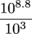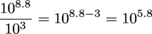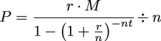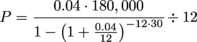Study Guide

# In the Real World

## In the Real World

Before we allow you to go forth and be completely radical, we have a serious ethical duty to connect you back to the real world from which you came. In other words, here's just a taste of what radicals and exponents might look like in the real world. Bon appétit.

### The Richter Scale

The Richter Scale, believe it or not, is measured using a logarithmic base of 10. Wait, what? A logarithmic who? Yes, we said it. The Richter Scale is measured using a logarithmic base of 10. Lucky for us, we can actually translate this to the world of exponents pretty easily.

All this base-10 business is really trying to say is that if California experiences an earthquake with a magnitude of 3 and Japan experiences a quake with a magnitude of 4, the Japan earthquake is actually 10 times stronger. An earthquake measuring a 5 on the Richter Scale would be 100 times stronger than one measuring a 3.

Now here's the question:

If people in Northern Sumatra, Indonesia experience an earthquake measuring a 3 on the Richter Scale and people in Melo, Uruguay experience an earthquake measuring an 8.8 on the Richter Scale (that's a big one), how much more intense would the Uruguay earthquake have been?

We can use our laws of exponents here, and things will work out very nicely. Since the Richter Scale is using a base of 10, we will too. If we say that a quake measuring a 3 has a factor of 103 and a quake with a factor of 4 is 10 times greater, we could actually measure this one at 104.

A 5 on the Richter Scale would translate to a 105 factor. So 8.8 would translate to 108.8. The question is, how many times greater is 108.8 than 103?

Subtracting 108.8 from 103 would give us the difference, but we want to know the multiple needed to go from 103 to 108.8. So let's divide.This is B-E-A-utiful because now we can apply our exponent rules.This means that the earthquake in Uruguay is 105.8 times more intense, or approximately 630,957 times worse. Yikes. Makes us feel bad for the people experiencing the imaginary earthquake in Uruguay.

### Rootin' Around

Let's say you're thinking of buying a house, and the realtor shows you one with an enormous backyard in the shape of a perfect square. It's even got a garden shed and a tire swing. Talk about idyllic.

The realtor tells you the backyard has an area of 1296 square feet. You want to know specifically how long each side of the yard is, since the fence is kinda sagging and you'll need to replace it eventually. But the realtor has no idea, and you don't have a ruler long enough to just straight-up measure it. How would you find the length of each side?

With a radical, that's how. Since the area of a square is A = s2, we can plug in what we know and track down s, the side length.

A = s2

1296 = s2

Now take the square root of both sides to get rid of that exponent.

√(1296) = √(s2)

√(1296) = s

Hmm...we're not sure what the square root of 1296 is, off the top of our heads. So let's split it up into factors. It looks like it might be divisible by 6.

√(1296) = s

√(6 × 216) = s

Beautiful. Let's keep going.

√(6 × 6 × 36) = s

√(6 × 6 × 6 × 6) = s

Hey, now we're cooking. We can stop right there; that's two pairs of 6s. The square root of (6 × 6) is just 6, so let's finish up.

√(6 × 6 × 6 × 6) = s

(6)(6) = s

36 = s

There we go. Each of the yard's sides is 36 feet long. Plenty of room for a pooch and a barbecue. And speaking of buying a house...

### For the Future Homeowner In All of Us

The loan a person takes out to pay for a home is typically called a mortgage. What's epically sweet about that is that the word comes from the French term meaning "death contract." Anyway, the way in which the mortgage is repaid is called an amortization schedule. Perhaps even better than the death contract reference is the fact that the amortization can be calculated using an exponential formula.In this equation, the variables are as follows:

P = Monthly Payment
r = Annual Interest Rate
M = Mortgage Amount
t = Number of Years
n = Number of Payments Per Year

So, for example, say we decided to purchase a home for \$200,000. We pick a 10% down payment at the time of sale. In other words, we've agreed to pay \$20,000 up front. This means we'll need to take out a loan or mortgage for the remaining \$180,000. If we make a few more assumptions, we can calculate our monthly payment.

P = Monthly Payment = What we want to find.
r = Annual Interest Rate = Let's say 4%... it's a buyer's market.
M = Mortgage Amount = \$180,000
t = Number of Years = 30...this is the typical mortgage length.
n = Number of Payments Per Year = 12...we're paying once a month.

Let's substitute and calculate.Instead of going through every step, we'll go out on a limb here and say you could handle this using a handy-dandy calculator. After all, no one in the real world is evaluating this monster by hand.

P ≈ 859.34753

This means that by rounding to the nearest cent, we now owe the bank \$859.35 every month for the next 30 years.

If we take this and multiply it by 12 months per year and then again by 30 years, we can find how much we'll actually pay for our new home.

859.34753 × 12 × 30 ≈ 309,365

Holy nuggets: \$309,365 plus our original \$20,000 down payment means we just paid \$329,365 for a house that originally cost \$200,000. The bank made, well, some serious bank.

We could do a lot of other great stuff with this equation too. For instance, we could start with the amount of money we had to spend each month and the known interest rate, then work backwards to how much we could spend on a house in the first place. Not a bad skill to have just from understanding a few exponents.